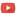KE8QZC | SFW | TSWSyllabus: [pdf] [tex]

Exams
Exam 1: [pdf] [tex]
Exam 2: [pdf] [tex]
Exam 3: [pdf] [tex]

Homework
Homework 1 (due 23 January) (solution): p.10: #1, 3 (explain why), 4, 7, 9, 15, 16; p.15: #2, 4, 6
Homework 2 (due 28 January) (solution): p.15: #2 (just draw slope field and nullcline(s) -- software is ok to use), 4, 6; p. 19: #1, 3, 4b, 4c, 9, 11; p. 26: #1a, 1b, 1c
Homework 3 (due 4 February) (solution): p. 26: #1d, 1h, 4a; p.32: #1, 3; p.34: #2, 4; p.41: #1, 2a, 2b, 3a, 3d
Homework 4 (due 11 February) (solution): p.41: #2d, 2e; p.90: #1a, 1b, 1c, 1d, 2 (do only 1a and 1c for #2), 3 (do only 1b and 1d for #3); p.94: #1a, 1b, 1c, 1d, 2 (do only 1a and 1c for #2); p.111: #2a, 2b, 2c
Homework 5 (due 20 February) (solution): p.120: #1c (it should be $t^2x''+3tx'+x=0$ -- there is a typo), 1e, 1f; p.124: #1a, 1e
Homework 6 (due 25 February) (solution): p.144: #3, 8a, 8b, 9a, 9c, 9d, 9j; p.156: #1, 6a, 6c, 6d,
Homework 7 (due 4 March) (solution): p.144: #8c, 8f, 9b, 9f; p.156: #6b, 6e, 14; p.162: #1b, 1c, 6, 7; p.173: #1, 2, 3
Homework 8 (due 27 March) (solution): p.190: #3a, 3c, 3d; p.198: #1, 2a, 3, 5, 6, 8
Homework 9 (due 3 April) (solution): p.198: #2 (note: $(1,2)^T = \begin{bmatrix} 1 \\ 2 \end{bmatrix}$), 3a, 6a, 8 (note: $(2,-3)^T=\begin{bmatrix} 2\\-3 \end{bmatrix}$ and $(-4,8)^T = \begin{bmatrix} -4 \\ 8 \end{bmatrix}$); p.202 #1a, 1c, 1e, 2 (do only 1a,1c,1e for this), 3; p.207: #1a, 1c, 1f, 1j, 1n
Homework 10 (due 10 April) (solution): p.207: #1b, 1d, 1e; p.218: #1, 2, 3
Homework 11 (due 15 April) (solution): p.221: #1, 2; p.225: #1; p.237: #1, 2, 4a, 4b, 4d, 4e, 5, 6
Homework 12 (due 3 May) (solution): p.257: #1a, 1b, 1c, 1d
Homework 13 (due 10 May) (solution): In this homework, we will use the Euler method (Section 6.2.1 in the text) to approximate the solution of the initial value problem $\left\{ \begin{array}{ll} x' = e^{-\sin(x)} \\ x(0)=-1 \end{array} \right.$ over the interval $[0,5]$.
1. Using $h=0.5$, approximate $x(0.5)$ and $x(1)$.
2. Using $h=0.25$, approximate $x(0.25)$ and $x(0.5)$.
3. Using $h=0.1$, approximate $x(0.1)$ and $x(0.2$.
4. Use a spreadsheet to plot the appoximations for each of the $h$'s above on the interval $[0,5]$. You should not expect to be able to find a "closed form solution" to this IVP.
BONUS (due 10 May): [pdf] [tex]

Quizzes
Quiz 1 (due 16 January): (solution) Given $v(t)=Ve^{-\frac{kt}{m}}$, show that $v$ solves the initial value problem $\left\{ \begin{array}{ll} -kv = mv' \\ v(0)=V \end{array} \right.$
Quiz 2 (due 23 January): (solution) Show that $x(t)=\dfrac{e^{2t}-1}{e^{2t}+1}$ solves $\left\{ \begin{array}{ll} x'&=1-x^2\\ x(0)&=0 \end{array} \right.$.
Quiz 3 (due 25 January): (solution) Solve the initial value problem $\left\{ \begin{array}{ll} x' = 2t \\ x(0)=5. \end{array} \right.$
Quiz 4
Quiz 5 (due 22 February): Compute the Laplace transform of $\mathscr{L}\{t^2\}(s)$ from the definition of $\mathscr{L}$.
Quiz 6 (due 20 March) (solution): Solve $\left\{ \begin{array}{ll} x' = -y \\ y'=x \\ x(0)=1, y(0)=0. \end{array} \right.$
Quiz 7 (due 25 March) (solution): Find the inverse of the matrix $A = \begin{bmatrix} 3&0 \\ 1&10\end{bmatrix}$ and show that it works properly by computing $AA^{-1}$.
Quiz 8 (due 1 April) (solution): In class we found the eigenvalues of $A=\begin{bmatrix} -2&-3\\3 & -2 \end{bmatrix}$ were $\lambda=-2\pm 3i$. We then showed that an eigenvector associated with $\lambda=-2+3i$ was $\vec{v}=\begin{bmatrix} 1\\ -i \end{bmatrix}$. Find an eigenvector associated with the other eigenvalue $\lambda=-2-3i$.
Quiz 9 (due 8 April) (solution): In class we showed that $\lambda=0$ and $\vec{v}=\begin{bmatrix} 1 \\ 2 \end{bmatrix}$ is an eigenpair for the matrix $A=\begin{bmatrix} 2&1 \\ 4&2 \end{bmatrix}$. Find the other eigenpair for this matrix.
Notes
1 May 2019: Euler method spreadsheet from class: here